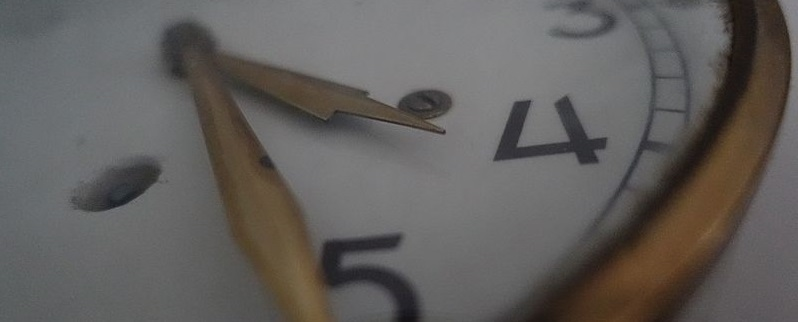# Sites2See – measurement for primary

Primary KLA:
Mathematics
Educational levels:
Foundation, Year 1, Year 2, Year 3, Year 4, Year 5, Year 6By Illymarry (Own work) [CC BY-SA 4.0], via Wikimedia Commons

Selected links to a range of interactive and print resources for Measurement topics in K-6 Mathematics.

## NSW syllabus outcomes

(MAe-9MG) describes and compares lengths and distances using everyday language

(MAe-10MG) describes and compares areas using everyday language

(MAe-11MG) describes and compares the capacities of containers and the volumes of objects or substances using everyday language

(MAe-12MG) describes and compares the masses of objects using everyday language

(MAe-12MG) describes and compares the masses of objects using everyday language

(MA1-9MG) measures, records, compares and estimates lengths and distances using uniform informal units, metres and centimetres

(MA1-10MG) measures, records, compares and estimates areas using uniform informal units

(MA1-11MG) measures, records, compares and estimates volumes and capacities using uniform informal units

(MA1-12MG) measures, records, compares and estimates the masses of objects using uniform informal units

(MA1-12MG) measures, records, compares and estimates the masses of objects using uniform informal units

(MAe-9MG) describes and compares lengths and distances using everyday language

(MAe-10MG) describes and compares areas using everyday language

(MAe-11MG) describes and compares the capacities of containers and the volumes of objects or substances using everyday language

(MAe-12MG) describes and compares the masses of objects using everyday language

(MAe-12MG) describes and compares the masses of objects using everyday language

(MA2-9MG) measures, records, compares and estimates lengths, distances and perimeters in metres, centimetres and millimetres, and measures, compares and records temperatures

(MA2-10MG) measures, records, compares and estimates areas using square centimetres and square metres

(MA2-11MG) measures, records, compares and estimates volumes and capacities using litres, millilitres and cubic centimetres

(MA2-12MG) measures, records, compares and estimates the masses of objects using kilograms and grams

(MA2-12MG) measures, records, compares and estimates the masses of objects using kilograms and grams

(MA3-9MG) selects and uses the appropriate unit and device to measure lengths andÂ distances, calculates perimeters, and converts between units of length

(MA3-10MG) selects and uses the appropriate unit to calculate areas, including areas of squares, rectangles and triangles

(MA3-11MG) selects and uses the appropriate unit to estimate, measure and calculate volumes and capacities, and converts between units of capacity

(MA3-12MG) selects and uses the appropriate unit and device to measure the masses of objects, and converts between units of mass

(MA3-12MG) selects and uses the appropriate unit and device to measure the masses of objects, and converts between units of mass

## Australian curriculum content descriptions

(ACMMG006) Use direct and indirect comparisons to decide which is longer, heavier or holds more, and explain reasoning in everyday language

(ACMMG007) Compare and order the duration of events using the everyday language of time

(ACMMG008) Connect days of the week to familiar events and actions

(ACMMG019) Measure and compare the lengths and capacities of pairs of objects using uniform informal units

(ACMMG020) Tell time to the half-hour

(ACMMG021) Describe duration using months, weeks, days and hours

(ACMMG037) Compare and order several shapes and objects based on length, area, volume and capacity using appropriate uniform informal units

(ACMMG038) Compare masses of objects using balance scales

(ACMMG039) Tell time to the quarter-hour, using the language of 'past' and 'to'

(ACMMG040) Name and order months and seasons

(ACMMG139) Interpret and use timetables

(ACMMG138) Connect volume and capacity and their units of measurement

(ACMMG137) Solve problems involving the comparison of lengths and areas using appropriate units

(ACMMG136) Convert between common metric units of length, mass and capacity

(ACMMG135) Connect decimal representations to the metric system

(ACMMG110) Compare 12- and 24-hour time systems and convert between them

(ACMMG109) Calculate the perimeter and area of rectangles using familiar metric units

(ACMMG108) Choose appropriate units of measurement for length, area, volume, capacity and mass

(ACMMG086) Use am and pm notation and solve simple time problems

(ACMMG085) Convert between units of time

(ACMMG290) Compare objects using familiar metric units of area and volume

(ACMMG041) Use a calendar to identify the date and determine the number of days in each month

(ACMMG061) Measure, order and compare objects using familiar metric units of length, mass and capacity

(ACMMG062) Tell time to the minute and investigate the relationship between units of time

(ACMMG084) Use scaled instruments to measure and compare lengths, masses, capacities and temperatures

Resource type:
Interactive Resource
Audience:
learner, teacher
ScOT topics:
Mass, Area, Distance, Time, Timetables, Length, Perimeter
File type:
text/html
Language/s:
en-AU
Author:
State of NSW, Department of Education
Publisher:
State of NSW, Department of Education
Date created:
Monday, 5 November 2018

Resource ID: bafe1016-ea01-414e-aac1-a70dc8319b72